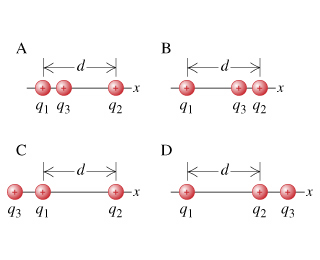# [SOLVED] Physics problem based on a picture, but hard!?

edited

Physics problem based on a picture, but hard!?

Two charged particles, with charges q_1=q and q_2=4q, are located at a distance d=2.00cm apart on the x axis. A third charged particle, with charge q_3=q, is placed on the x axis such that the magnitude of the force that charge 1 exerts on charge 3 is equal to the force that charge 2 exerts on charge 3.

Find the position of charge 3 when q = 1.00 nC.

The question is: Which of the following sketches represents a possible configuration for this problem?

Based on this picture:by

q3 must be closer to q1 than to q2 for the force magnitudes to be the same, simply because q2 has 4 times the charge as q1. Therefore, B and D are eliminated right off.

The question acts about the MAGNITUDE of the force that q1 and q2 exert on q3; we don't have to worry about the direction of the force. The magnitudes are given by q1q3/(r13)^2 and q2q3/(r23)^2, but we know that q1q3 is 1/4 what q2q3 is. Therefore, r23 must be twice what r13 is, to make these magnitudes the same. In math-speak:

q*q/(r13)^2 = q*4q/(r23)^2, so that 1/(4r13^2) = 4/(4r23^2)

==> r23^2 = 4r13^2

==> r23 = 2*r13.

We have two possibilities: q3 is between q1 and q2, or (reading left-to-right) their positions are q3, q1, q2.

q3 Between:

We know that r12 = 2.00 cm. Also, r13 + r23 = r12, so that r13 + 2*r13 = 2 cm, and so r13 = 0.66 cm. Here, q3 is 1/3 of the way between q1 and q2.

q3 to the left:

We know that r12 = 2.00 cm. Also, r13 + r12 = r23, so that r13 + 2 cm = 2 r13. Thus, r13 = 2cm (the same distance as r12).

From the diagrams given, if they're anything to scale, the only possible answer is (A), as in (C), r13 is obviously quite a bit less than r12. If they're NOT drawn to scale, then both (A) and (C) could be answers.

Lorem ipsum dolor sit amet, consectetur adipiscing elit, sed do eiusmod tempor incididunt ut labore et dolore magna aliqua. Ut enim ad minim veniam, quis nostrud exercitation ullamco laboris nisi ut aliquip ex ea commodo consequat. Duis aute irure dolor in reprehenderit in voluptate velit esse cillum dolore eu fugiat.

+1 vote